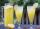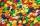Product of two fractions

Product of two fractions is 9 3/5 . If one of the fraction is 9 3/7. Find the other fraction.

Result

a =  1.018

Solution:Leave us a comment of example and its solution (i.e. if it is still somewhat unclear...):Be the first to comment!To solve this example are needed these knowledge from mathematics:

Need help calculate sum, simplify or multiply fractions? Try our fraction calculator.

Next similar examples:

1. Unit rateFind unit rate: 6,840 customers in 45 days
2. Fraction to decimalWrite the fraction 3/22 as a decimal.
3. Mixed2improperWrite the mixed number as an improper fraction. 166 2/3
4. Fraction and a decimalWrite as a fraction and a decimal. One and two plus three and five hundredths
5. ZdeněkZdeněk picked up 15 l of water from a 100-liter full-water barrel. Write a fraction of what part of Zdeněk's water he picked.
6. Write 2Write 791 thousandths as fraction in expanded form.
7. Fractions 4How many 2/3s are in 6?
8. Pizza 5You have 2/4 of a pizza and you want to share it equally between 2 people how much pizza does each person get?How many 1/4 cup servings are in 2 and 1/3 cups of lemonade?
10. Cake 71/3 of a cake shared with 4 people. What share of the whole cake has each people?
11. ChocolateChildren break chocolate first to third and then every part of another half. What kind got each child? Draw a picture. What part would have received if each piece have halved?
12. In fractionsAn ant climbs 2/5 of the pole on the first hour and climbs 1/4 of the pole on the next hour. What part of the pole does the ant climb in two hours?
13. Lengths of the poolMiguel swam 6 lengths of the pool. Mat swam 3 times as far as Miguel. Lionel swam 1/3 as far as Miguel. How many lengths did mat swim?
14. CakesOn the bowl were a few cakes. Jane ate one-third of them, Dana ate a quarter of those cakes that remained. a) What part (of the original number of cakes) Dana ate? b) At least how many cakes could be (initially) on thebowl?
15. Ratio 11Simplify this ratio 10 : 1/4
16. TeacherTeacher Rem bought 360 pieces of cupcakes for the outreach program of their school. 5/9 of the cupcakes were chocolate flavor and 1/4 wete pandan flavor and the rest were vanilla flavor. How much more chocolate flavor cupcakes than vanilla flavor?
17. SweetsMom bought box of sweets for their children.Whole package of 100 sweets divided among 4 their children so that each child receives the most and for she remains the least sweets. How many sweets left for mom.